A page replacement algorithm determines how the victim page (the page to be replaced) is selected when a page fault occurs. The aim is to minimize the page fault rate.

The efficiency of a page replacement algorithm is evaluated by running it on a particular string of memory references and computing the number of page faults.

Reference strings are either generated randomly, or by tracing the paging behavior of a system and recording the page number for each logical memory reference.

The performance of a page replacement algorithm is evaluated by running it on a particular  string of memory references and computing the number of page faults.

Consecutive references to the same page may be reduced to a single reference, because they won’t cause any page fault except possibly the first one:

(1,4,1,6,1,1,1,3) -> (1,4,1,6,1,3)

We have to know the number of page frames available in order to be able to decide on the page replacement scheme of a particular reference string. Optionally, a frame allocation policy may be followed.

1 Optimal Page Replacement Algorithm (OPT)
In this algorithm, the victim is the page which will not be used for the longest period. For a fixed number of frames, OPT has the lowest page fault rate between all of the page replacement algorithms, but there is problem for this algorithm. OPT is not possible to be implemented in practice. Because it requires future knowledge. However, it is used for performance comparison.

Example
Assume we have 3 frames and consider the reference string below.
Reference string: 5, 7, 6, 0, 7, 1, 7, 2, 0, 1, 7, 1, 0
Show the content of memory after each memory reference if OPT page replacement algorithm is used. Find also the number of page faultsAccording to the given information, this algorithm generates a page replacement scheme with 7 page faults.

2 First-In-First-Out (FIFO)
This is a simple algorithm, and easy to implement. The idea is straight forward: choose the oldest page as the victim.

Example
Assume there are 3 frames, and consider the reference string given in example . Show the content of memeory after each memory reference if FIFO page replacement algorithm isused.

Find also the number of page faults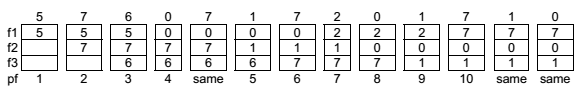10 page faults are caused by FIFO

Normally, one would expect that with the total number of frames increasing, the number of page faults decreases. However, for FIFO, there are cases where this generalization fails. This is called Belady’s Anomaly.

As an exercise consider the reference string below. Apply the FIFO method and find the number of page faults considering different number of frames. Then, examine whether the replacement suffer Belady’s anomaly.
Reference string: 1, 2, 3, 4, 1, 2, 5, 1, 2, 3, 4, 5

3 Least Recently Used (LRU)
In this algorithm, the victim is the page that has not been used for the longest period. So, this algorithm makes us be rid of the considerations when no swapping occurs.

The OS using this method, has to associate with each page, the time it was last used which means some extra storage. In the simplest way, the OS sets the reference bit of a page to “1” when it is referenced. This bit will not give the order of use but it will simply tell whether the corresponding frame is referenced recently or not. The OS resets all reference bits periodically

Example
Assume there are 3 frames, and consider the reference string given in example . Show the content of memory after each memory reference if LRU page replacement algorithm is used. Find also the number of page faults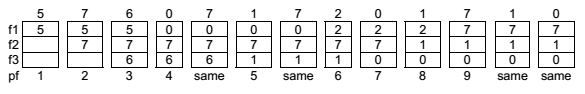This algorithm resulted in 9 page faults.

Frame Allocation
In order to be able to decide on the page replacement scheme of a particular reference string, we have to know the number of page frames available In page replacement, some frame allocation policies may be followed.

• Global Replacement: A process can replace any page in the memory.
• Local Replacement: Each process can replace only from its own reserved set of allocated page frames. In case of local replacement, the operating system should determine how many frames should the OS allocate to each process.

The number of frames for each process may be adjusted by using two ways:

1. Equal Allocation: If there are n frames and p processes, n/p frames are allocated to each process.
2. Proportional Allocation: Let the virtual memory size for process p be v(p). Let there are m processes and n frames. Then the total virtual memory size will be: V = Σv(p). Allocate (v(p) /V) * n frames to process p.

Example
Consider a system having 64 frames and there are 4 processes with the following virtual memory sizes: v(1) = 16, v(2) = 128, v(3) = 64 and v(4) = 48.

Equal Allocation: Assume that there are n frames, and p processes, then n/p frames are allocated to each process allocates 64 / 4 = 16 frames to each process.

Proportional Allocation: V = 16 + 128 + 64 + 48 = 256. It allocates:
(16 / 256) * 64 = 4 frames to process 1,
(128 / 256) * 64 = 32 frames to process 2,
(64 / 256) * 64 = 16 frames to process 3,
(48 / 256) * 64 = 12 frames to process 4.

Thrashing
A process is thrashing if it is spending more time for paging in/out (due to frequent page faults) than executing.

Thrashing causes considerable degradation in system performance. If a process does not have enough number of frames allocated to it, it will issue a page fault. A victim page must be chosen, but if all pages are in active use. So, the victim page selection and a new page replacement will be needed to be done in a very short time. This means another page fault will be issued shortly, and so on and so forth.

In case a process thrashes, the best thing to do is to suspend its execution and page out all its pages in the memory to the backing store.

Local replacement algorithms can limit the effects of thrashing. If the degree of multiprogramming is increased over a limit, processor utilization falls down considerably because of thrashing.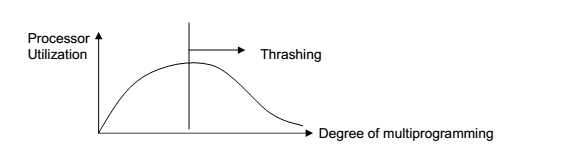To prevent thrashing, we must provide a process as many frames as it needs. For this, a model called the working set model is developed which depends on the locality model of program execution. But here we only mention its name and skip its details to limit the scope

Working Set Model
To prevent thrashing, we must provide a process as many frames as it needs. For this, we shall use the working set model, which depends on the locality model of program execution, discussed earlier.
We shall use a parameter, ∆, called the working set window size. We shall examine the last ∆ page references.
The set of pages in the last page references shall be called the working set of a process.

Example  : Assume ∆ = 10 , and consider the reference string given below, on which the window is shown at difeferent time instants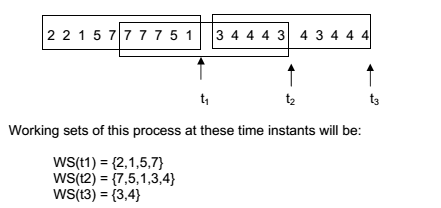Note that in calculating the working sets, we do not reduce consequent references to the same page to a single reference. Choice of ∆ is crucial. If ∆ is to small, it will not cover the entire working set. If it is too large, several localities of a process may overlap. Madnick and Donovan suggested ∆ to be about 10.000 references.

Now, compute the WS size (WSS) for each process, and find the total demand, D of the system at that instance in time, as the summation of all the WS sizes.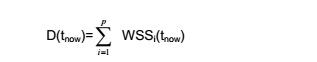If the number of frames is n, then
a. If D > n , the system is thrashing.
b. If D < n, the system is all right, the degree of multi-programming can possibly be increased. In order to be able to use the working set model for virtual memory management, the OS keeps track of the WS of each process. It allocates each process enough frames to provide it with its WS. If at one point D > n, OS selects a process to suspend. The frames that were used by the selected process are reallocated to other processes.

We can also use the page fault frequency to decide on decreasing or increasing the no. of frames allocated to a process.